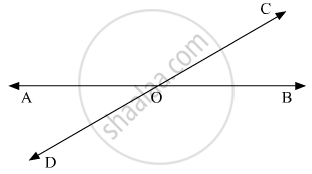# Two Lines Ab and Cd Intersect at O. If ∠Aoc + ∠Cob + ∠Bod = 270°, Then ∠Aoc = - Mathematics

MCQ

Two lines AB and CD intersect at O. If ∠AOC + ∠COB + ∠BOD = 270°, then ∠AOC =

• 70°

• 80°

• 90°

• 180°

#### Solution

Let us draw the following diagram showing two lines AB and  CD intersecting at a point O.Thus, ∠AOC, ∠COB, ∠AODand   ∠BODform a complete angle, that is the sum of these four angle is 360°.

That is,

∠AOC + ∠COB +∠BOD+ ∠AOD = 360°   (I)

It is given that

∠AOC + ∠COB + ∠BOD = 270°   (II)

Subtracting (II) from (I), we get:

∠AOD = 360° - 270°

∠AOD = 90°
If one of the four angles formed by two intersecting lines is a right angle, then each of the four angles will be a right angle.
So, ∠AOC = $90^\circ$

Concept: Concept of Parallel Lines
Is there an error in this question or solution?

#### APPEARS IN

RD Sharma Mathematics for Class 9
Chapter 10 Lines and Angles
Q 12 | Page 53